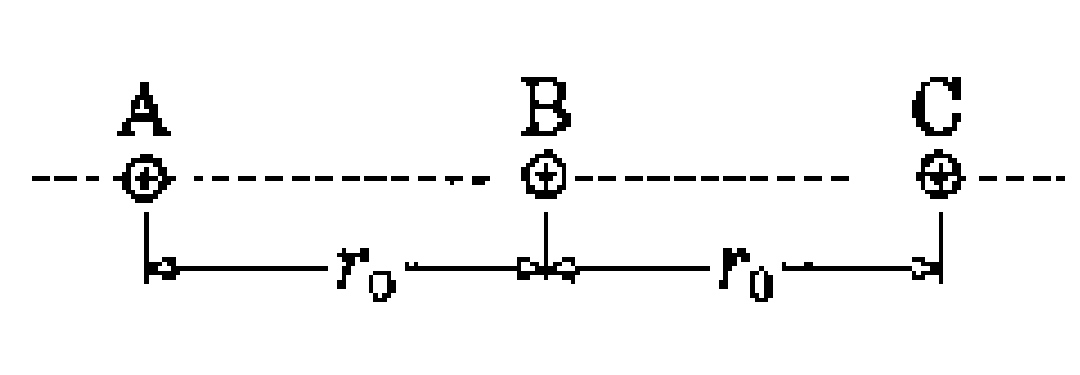# Electrostatics corrected version

Three particles $A$, $B$ and $C$ of charges $q$, $q$ and $2q$ and masses $m$, $2m$ and $5m$ respectively are held in free space in a straight line at separation $r_{0}$.
between two adjacent particles as shown in the figure. All the three particles are released simultaneously. Find velocities of all the three particles when they reach so far from each other that electrostatic interactions between them can be neglected.Type your answer as $v_{A}v_{B}v_{C}=?$

Details and Assumptions
1)$q=1$
2)$\epsilon_{0}=1$
3)$m=1$
4)$r_{0}=1$

×